A barplot is a graphical representation of data points in a dataset, where individual data points are represented by rectangular bars on a two-dimensional coordinate system. This type of plot allows us to visualize the distribution of categorical data by showing the frequency or count of each category along the plot.
Pandas, a powerful data manipulation library in Python, allow us to create easily scatter plots: check this introduction to barplots with pandas. In this post, we will explore how to leverage `Pandas` to customize barplots, making it good looking and studying available options.

## Libraries

Pandas is a popular open-source Python library used for data manipulation and analysis. It provides data structures and functions that make working with structured data, such as tabular data (like `Excel` spreadsheets or `SQL` tables), easy and intuitive.

To install Pandas, you can use the following command in your command-line interface (such as `Terminal` or `Command Prompt`):

`pip install pandas`

Matplotlib functionalities have been integrated into the pandas library, facilitating their use with `dataframes` and `series`. For this reason, you might also need to import the matplotlib library when building charts with Pandas.

This also means that they use the same functions, and if you already know Matplotlib, you'll have no trouble learning plots with Pandas.

``````import pandas as pd
import matplotlib.pyplot as plt``````

## Dataset

In order to create graphics with Pandas, we need to use pandas objects: `Dataframes` and `Series`. A dataframe can be seen as an `Excel` table, and a series as a `column` in that table. This means that we must systematically convert our data into a format used by pandas.

Since barplots need qualitative variables, we will create a dataset with a categorical variable.

``````# Create a list with 3 different categories
category = ['Group1']*30 + ['Group2']*50 + ['Group3']*20

# Store the data into a dataframe
df = pd.DataFrame({'category': category})``````

## Basic barplot

Once we've opened our dataset, we'll now create the graph. An important feature of barplots in Python is that you can't simply give them a column name as an argument.

Instead, we have to calculate the counts for each category ourselves and give them as input to the function. To do this, we simply use the pandas `value_counts()` function.

``````# Get the repartition of each category
values = df['category'].value_counts()

# Create the plot
values.plot.bar(grid=True) # Add a grid in the background
plt.show()``````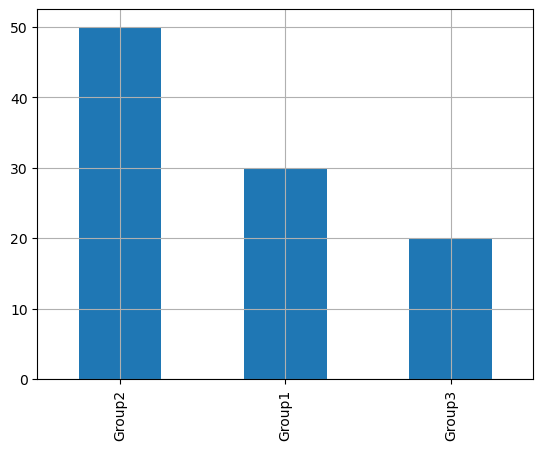## Change bar order

To change the order of the bars, we need to do 2 simple things:

• set the desired order in a list
• use the `reindex()` function to apply this order to `values`
``````# Get the repartition of each category
values = df['category'].value_counts()

# Define the desired order of categories
desired_order = ['Group1', 'Group2', 'Group3']  # Change this order as needed
values = values.reindex(desired_order) # Reindex the values in the desired order

# Create the plot
values.plot.bar(grid=True) # Add a grid in the background
plt.show()``````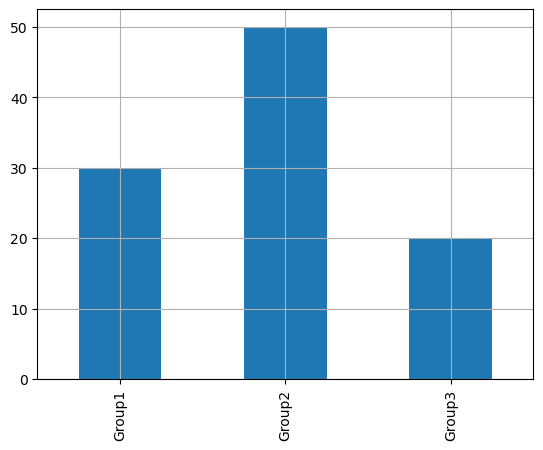## Change axis

If you want to change the axis of the bars, simply use the `barh()` function with the exact same arguments!

``````# Get the repartition of each category
values = df['category'].value_counts()

# Create the plot as a horizontal bar plot
values.plot.barh(grid=True)  # Use barh for a horizontal bar plot
plt.show()``````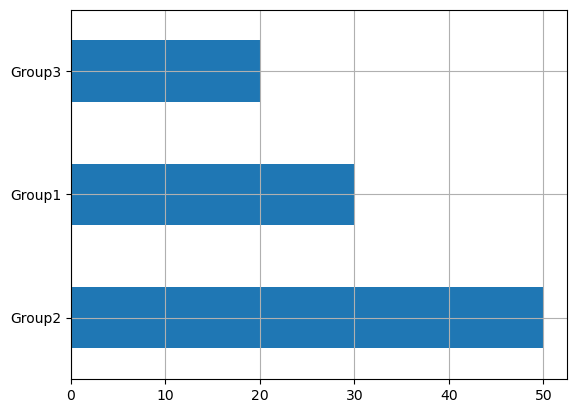## Custom color

In the `color` argument, you can put either one color (like `'red'`) or a list of colors (check below). The list must have the same length as the number of different categories

``````# Get the repartition of each category
values = df['category'].value_counts()

# Define colors to use
colors = ['#69b3a2', '#cb1dd1', 'palegreen']

# Create the plot as a horizontal bar plot
values.plot.bar(color=colors,
grid=True)  # Use barh for a horizontal bar plot
plt.show()``````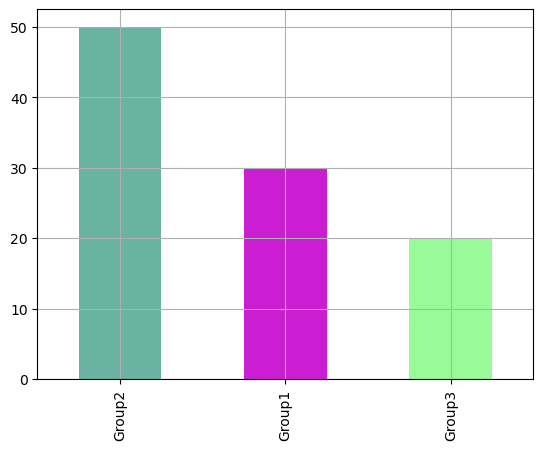## All together with a custom layout

If we put everything together, we can have a nice barplot built with Pandas

``````# Get the repartition of each category
values = df['category'].value_counts()

# Define the desired order of categories
desired_order = ['Group1', 'Group2', 'Group3']  # Change this order as needed
values = values.reindex(desired_order) # Reindex the values in the desired order

# Define colors to use
colors = ['#69b3a2', '#cb1dd1', 'palegreen']

# Create the plot as a horizontal bar plot
ax = values.plot.barh(color=colors,
grid=True,
)

ax.set_title('Customized Barplot')
ax.set_xlabel('Numbers by category')

plt.legend()
plt.show()``````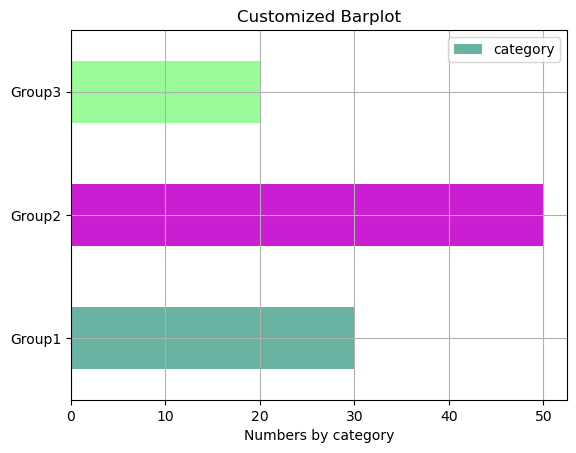## Going further

This post explains how to customize title, axis and markers of a barplot built with pandas.

For more examples of how to create or customize your plots with Pandas, see the pandas section. You may also be interested in how to customize your barplots with Matplotlib and Seaborn.

## Contact & Edit

👋 This document is a work by Yan Holtz. You can contribute on github, send me a feedback on twitter or subscribe to the newsletter to know when new examples are published! 🔥